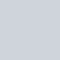52. N-Queens II

hard

# 個人範例程式碼

``````class Solution:
def totalNQueens(self, n: int) -> int:
if n == 0:
return [[]]

self.ans = 0
self.used_horizontal = set()
self.used_diagonal_x_minus_y = set()

board_set = set()
self.dfs(n, board_set, 0)
return self.ans

def dfs(self, n, board_set, floor):
# end of recursion
if floor == n:
self.ans += 1
return

# define and split
for i in range(n):
queen = (floor, i)
if self.check_board_conditions(queen):
self.dfs(n, board_set, floor+1)
self.remove_board_conditions(queen) # backtracking
board_set.remove(queen) # backtracking

def check_board_conditions(self, queen):
(floor, i) = queen
if i in self.used_horizontal:
return False
if floor + i in self.used_diagonal_x_add_y:
return False
if floor - i in self.used_diagonal_x_minus_y:
return False
return True

def remove_board_conditions(self, queen):
(floor, i) = queen
self.used_horizontal.remove(i)
self.used_diagonal_x_minus_y.remove(floor - i)

(floor, i) = queen
``````

## 算法說明

• 此題目的前一題要求列出全部結果，而這題只須回傳結果數量，但做法相同，前一題可參考：

【Leetcode】python –  N-Queens 個人解法筆記

## board 正確的判斷條件

• 橫的不重複
• 直的不重複
• 斜的不重複

### 橫的不重複

• 橫的不重複：我們在 for 環圈的時候，一橫只允許一個，因此不用另外判斷

### 直的不重複

• 直的不重複：我們紀錄直的座標到 used，每次嘗試擺上一個新皇后時，看一下有沒有 used

### 斜的不重複

• 斜的不重複：這個比較複雜，基本還是使用 used 的概念，細節我們下面細講

• 正斜線：x+y
• 負斜線：x-y• 正斜線：x+y，範圍介於 0 ~ 2n
• 負斜線：x-y，範圍介於 -n ~ n# Reference